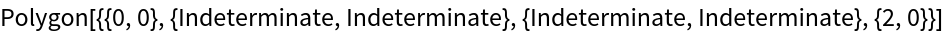#Function Repository Resource:

# Trapezoid

Create quadrilateral polygon with two parallel sides

Contributed by: Nikolay Murzin  |  N. Murzin
 ResourceFunction["Trapezoid"][a,α,b,β] represents a filled trapezoid with parallel sides with lengths a and b, and base angles α and β.

## Details and Options

The parameters a, b, α and β used in ResourceFunction["Trapezoid"] are as shown in the following diagram: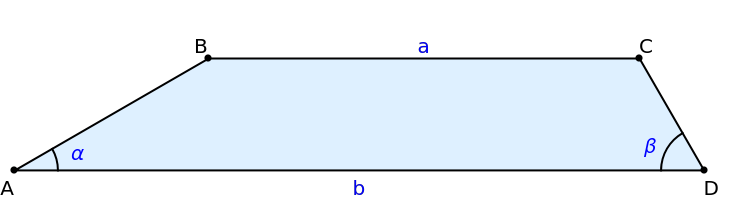ResourceFunction["Trapezoid"] returns a Polygon with A at the origin and D on the positive x-axis.
The shorter of the parallel sides of the trapezoid is always on top. With a<b, line BC lies in the half-plane y>0; otherwise, it lies in the y<0 half-plane.

## Examples

### Basic Examples (3)

A trapezoid with sides 2 and 6 and base angles of 60° and 30°:

 In:=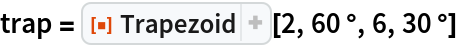Out=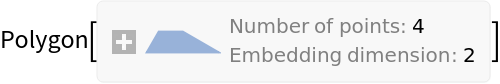Visualize it:

 In:=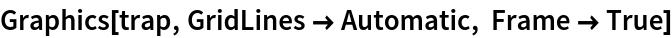Out=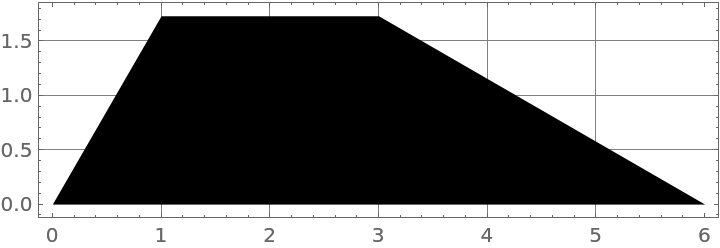Compute the area of this shape:

 In:=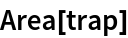Out=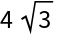### Scope (1)

Get symbolically-computed coordinates for the vertices of a trapezoid:

 In:=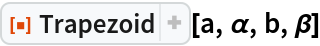Out=### Applications (1)

Use a trapezoid in a GeometricScene computation:

 In:=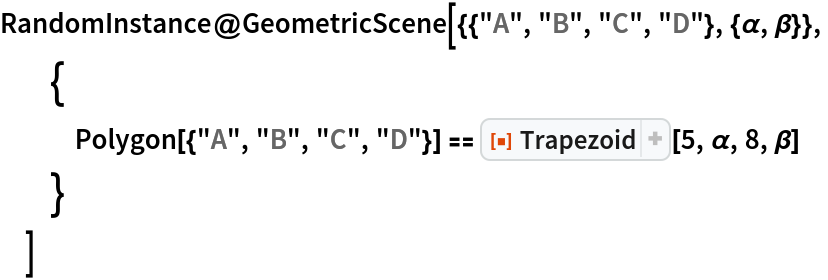Out=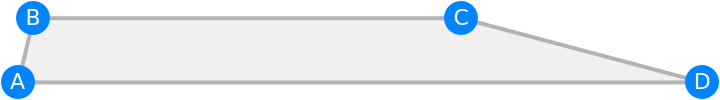### Properties and Relations (1)

A trapezoid with a base side of length 0 may represent a triangle:

 In:=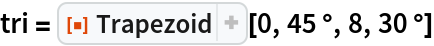Out=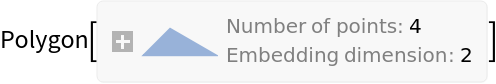In:=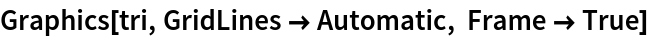Out=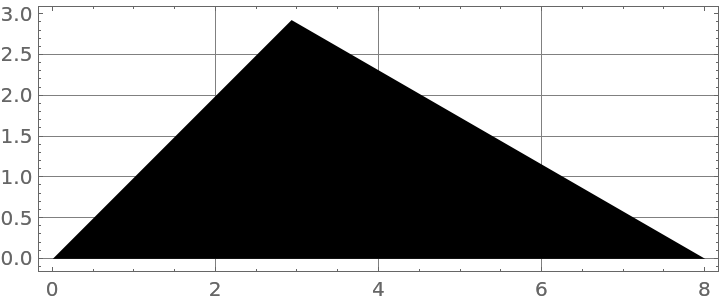### Possible Issues (3)

A trapezoid with equal sides is ambiguous; therefore, it degenerates into a line:

 In:=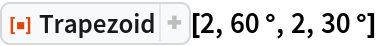Out=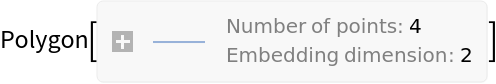A trapezoid with parallel left and right sides stretches out to infinity:

 In:=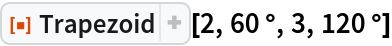Out=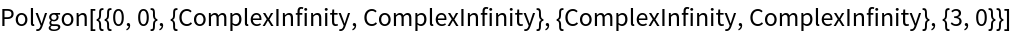A trapezoid with equal base sides and parallel left-right sides is indeterminate:

 In:=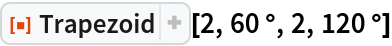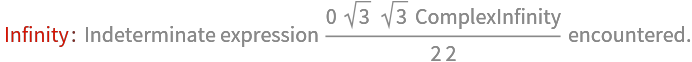Out=